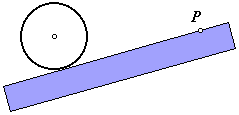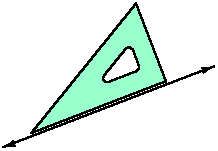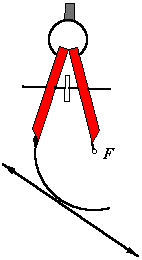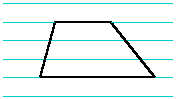# What is a construction?

Geometric constructions go back to Greek antiquity. They are often called Euclidean constructions, but they certainly predate Euclid. The phrase compass and straightedge construction may be more descriptive. Those are the only instruments allowed. The compass establishes equidistance, and the straightedge establishes collinearity. All geometric constructions are based on those two concepts.

The compass does not simply draw curves. It cannot be replaced by a circle template or a coffee can. The compass is anchored at a center point, and keeps the pencil at a fixed distance from that point. All points on the curve drawn by a compass are equidistant from the center point.

Although rulers are often used as straightedges, the graduation marks may not be used. No measurements are allowed. The straightedge is used only for drawing lines. Given any two distinct points, this instrument can draw the set of all points that are collinear with them.

Rulers and protractors have their place in geometry, but these are not construction instruments. All measurements are approximations. In reality, the compass and straightedge also are subject to error. A line has zero width, but a drawing of a line does not. However, construction is a theoretical exercise. If we were not limited by the physical imperfections of the instruments, the drawing surface, and the person using them, then construction instruments would render exact results. This is not true of measurement instruments.

### Practices to Avoid

I once used a ukulele to stir a beer wort. That did not make me a musician (or a brewer, as it turned out). The point is, using construction instruments is not sufficient for making your drawing a construction. Here are some guidelines and common mistakes.

 To construct a line, a ray, or a line segment, the straightedge must be aligned across exactly two fixed points. This rule cannot be ignored, unless it is a case in which the position or direction of the line is arbitrary. In this figure, the objective is to construct a line through point P, tangent to the circle. The straightedge has been placed on point P, and is being rotated into position. This is not a construction. The direction of the line cannot be established by approximating tangency. Another point must be constructed.Drafting triangles are often used as straightedges. This practice is acceptable, but do not use the angles as templates. In this figure, a drafting triangle is being used to draw a perpendicular line. This is not a construction. In essence, the instrument is being used to measure a 90° angle, just as a protractor would be used.Here is another misguided attempt to approximate tangency. This time it is with a compass. When constructing curves (other than arbitrary curves), the compass must have a fixed center point, and the radius must be set by the distance between two fixed points. In the figure at right, the radius is only a guess. Another point must be constructed to set the radius.I once gave an assignment in which the students were to construct a trapezoid (a quadrilateral with one pair of parallel sides). Many of them turned in something like this. Having ruled paper can be convenient for sketching, but do not mistake this for a construction. The students were using the paper itself as an instrument. On this same note, do not lay a ruler down and draw along both edges in order to make parallel lines. That is not construction either.### The Collapsing Compass

In many commonly accepted constructions (e.g., congruent angles), the compass radius is set by the distance between two points, and then the compass is centered on some third point, elsewhere on the drawing. Under the strictest traditional rules (thank the Greeks again), this was not allowed. The radius was established only by the distance from the center point to some second fixed point. It was assumed that the moment the compass point was lifted, the instrument collapsed, making it impossible to retain the radius.

The constructions in this site ignore the collapsing compass rule. Given two points for the radius and a center point in the same plane, however distant, it is possible to draw the arc, even under the old rules. The construction for transferring the radius is elementary, but rather tedious. There is no clear purpose for the rule other than torturing students. Modern geometry classes can accomplish that with fifty-pound hall passes.

Last update: September 4, 2003 ... Paul Kunkel whistling@whistleralley.com
For email to reach me, the word geometry must appear in the body of the message.